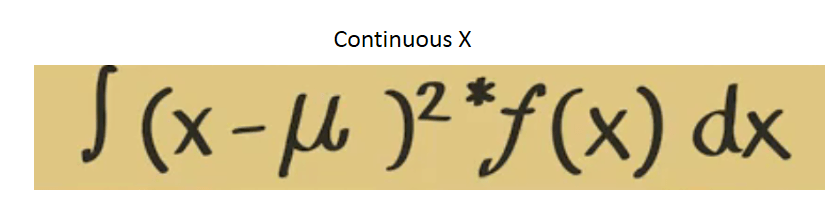### Random variables and probability distributions

#### Random variables:

A random variable is a function that associates a unique numerical value with every outcome of an experiment. The value of the random variable will vary from trial to trial as the experiment is repeated. The outcome of an experiment need not be a number, for example, the outcome when a coin is tossed can be ‘heads’ or ‘tails’. However, we often want to represent outcomes as numbers.

Let X represent a function that associates a Real number with each and every elementary event in some sample space S. Then X is called a random variable on the sample space S.

• If random variable can only equal a finite number of values, it is a discrete random variable. Probability distribution is known as a “probability mass function” or just p.m.f.
• If a random variable can equal an infinite (or really really large) number of values, then it is a continuous random variable. Probability distribution is know as a “probability density function” or just p.d.f.
• A probability distribution can be shown using tables or graph or mathematical equation.
##### Examples:
• A coin is tossed ten times. The random variable X is the number of tails that are noted. X can only take the values 0, 1, …, 10, so X is a discrete random variable.
• Examples of discrete random variables include the number of children in a family, the Friday night attendance at a cinema, the number of patients in a doctor’s surgery, the number of defective light bulbs in a box of ten.
• A light bulb is burned until it burns out. The random variable Y is its lifetime in hours. Y can take any positive real value, so Y is a continuous random variable.
• Examples of continuous random variables include height, weight, the amount of sugar in an orange, the time required to run a mile.

#### Probability Mass Function:

The probability distribution of a discrete random variable is a list of probabilities associated with each of its possible values. It is also sometimes called the probability function or the probability mass function.

More formally, the probability distribution of a discrete random variable X is a function which gives the probability p(xi) that the random variable equals xi, for each value xi:

p(xi) = P(X=xi)

It satisfies the following conditions:

• 0 <= p(xi) <= 1
• sum of all p(xi) is 1

This same concept was discussed in the previous page at the time of explaining Sample Space example with  one and two coin tosses. A probability distribution lists all possible outcome in the sample space and the probabilities with which they occur has given below.As, sum of probability of all outcomes add up to 1 so, it is called probability mass function or p.m.f.

I already mentioned that a probability distribution can be shown using tables or graph or mathematical equation. If we plot the same distribution using a histogram then X axis represents the cases (HH, TT, HT, TH)  and Y- axis represents it probability value. But for probability density functions (p.d.f.) Y-axis presents the probability density value. In the below picture a probability distribution of a lottery spinner has been shown.If we draw a histogram for this then it will look like this. Observed the X and Y axis.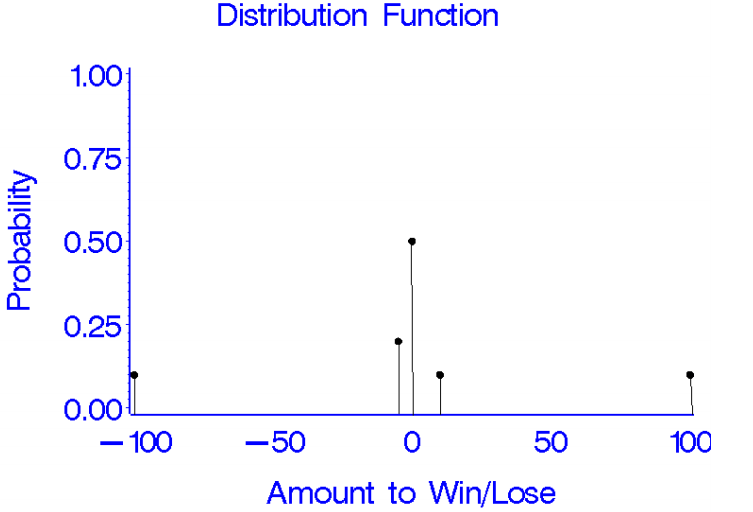#### Cumulative Distribution Function:

All random variables (discrete and continuous) have a cumulative distribution function. It is a function giving the probability that the random variable X is less than or equal to x, for every value x.

Formally, the cumulative distribution function F(x) is defined to be:

F(x) = P(X<=x)

for

-infinity < x < infinity

For a discrete random variable, the cumulative distribution function is found by summing up the probabilities as in the example below.

For a continuous random variable, the cumulative distribution function is the integral of its probability density function.

Example

Discrete case : Suppose a random variable X has the following probability distribution p(xi):

xi                             0              1              2              3              4              5

p(xi)                       1/32       5/32       10/32     10/32     5/32       1/32

This is actually a binomial distribution: Bi(5, 0.5) or B(5, 0.5). The cumulative distribution function F(x) is then:

xi                             0              1              2              3              4              5

F(xi)                       1/32       6/32       16/32     26/32     31/32     32/32

F(x) does not change at intermediate values. For example:

F(1.3) = F(1) = 6/32

F(2.86) = F(2) = 16/32

Consider another distribution example below. Now based on below picture what is the probability that X takes a value of either 2 or 3.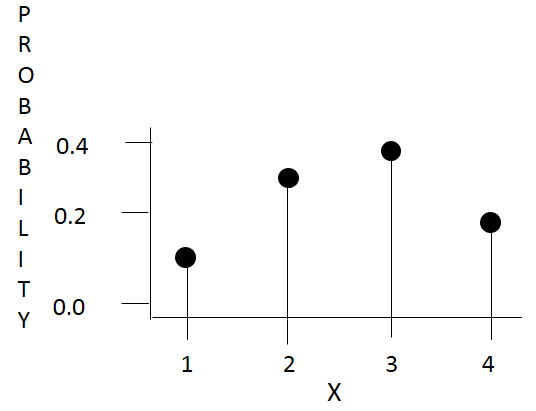P( X=2 or X=3 ) = P(X=2) + P(x=3) = 0.3+0.4 =0.7

Here union of probabilities = sum of probabilities.

What is the probability that X is greater than 1 ?

P(X=2 or X=3 or X=4 ) = 0.3+0.4+ 0.2 =0.9

On the other way you can tell P(X=2 or X=3 or X=4 )= 1- P(x=1) = 1- 0.1 = 0.9 both the answer are same. Here we are finding from complementary rule.

Based on a probability distribution we can easily calculate probabilities for values that are less than or equal to a given value. The probability of X is less than or 1 is 0.1. Similarly, probability of X is less than or equal to 2 is (0.1+0.3) =0.4 and so on.Probability histogram  of cumulative probability distribution has shown below for the above example.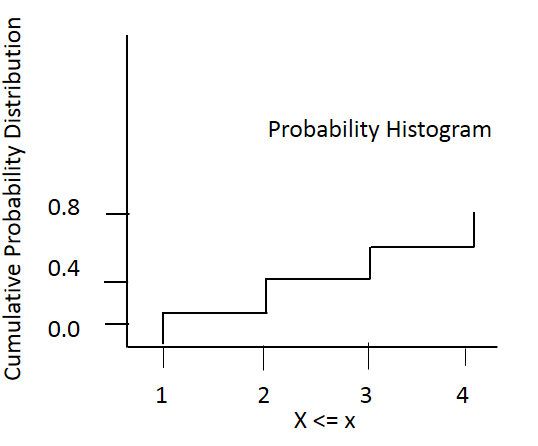#### Probability Density Function:

The probability density function of a continuous random variable is a function which can be integrated to obtain the probability that the random variable takes a value in a given interval. A probability density function will look like the below diagram. For p.d.f. Y-axis does represent the probability rather it represents the probability density value. To get probability from here you need to consider certain interval under the curve rather than height of the curve at certain location what we do for probability mass function. Here probability if given by the surface area under the curve with a interval.

More formally, the probability density function, f(x), of a continuous random variable X is the derivative of the cumulative distribution function F(x):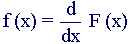Since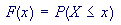it follows that: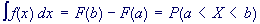Since F(x) = P(X<=x) If f(x) is a probability density function then it must obey two conditions:

• that the total probability for all possible values of the continuous random variable X is 1:
•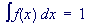• that the probability density function can never be negative: f(x) > 0 for all x.

#### Mean of a random variable:

The mean of a random variable indicates its average or central value. It is a useful summary value of the variable’s distribution.

As it is the expected average outcome from many observation so we can tell it as Expected value also.

Stating the expected value gives a general impression of the behavior of some random variable without giving full details of its probability distribution (if it is discrete) or its probability density function (if it is continuous).

• The mean of a discrete random variable is the probability-weighted average of all possible values that the random variable can take. Check the below formula for mean of discrete random variable.
• The mean of a continuous random variable is the average of all possible values that the random variable can take. We can represent that as integral and function of X. Check the formula for mean of a continuous random varibale.

The expected value of a random variable X is symbolized by E(X) or µ.

If X is a discrete random variable with possible values x1, x2, x3, …, xn, and p(xi) denotes P(X = xi), then the expected value of X is defined by:where the elements are summed over all values of the random variable X.

If X is a continuous random variable with probability density function f(x), then the expected value of X is defined by: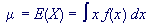##### Example:

For Discrete: When a die is thrown, each of the possible faces 1, 2, 3, 4, 5, 6 (the xi’s) has a probability of 1/6 (the p(xi)’s) of showing. The expected value of the face showing is therefore:

µ = E(X) = (1 x 1/6) + (2 x 1/6) + (3 x 1/6) + (4 x 1/6) + (5 x 1/6) + (6 x 1/6) = 3.5

Notice that, in this case, E(X) is 3.5, which is not a possible value of X.

#### Variance of a random variable:

The variance of a random variable is a non-negative number which gives an idea of how widely spread the values of the random variable are likely to be; the larger the variance, the more scattered the observations on average.

Stating the variance gives an impression of how closely concentrated round the expected value the distribution is; it is a measure of the ‘spread’ of a distribution about its average value.

Variance is symbolized by V(X) or Var(X) or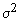The variance of the random variable X is defined to be:where E(X) is the expected value or mean  of the random variable X.

• The larger the variance, the further that individual values of the random variable (observations) tend to be from the mean, on average;
• the smaller the variance, the closer that individual values of the random variable (observations) tend to be to the mean, on average;
• taking the square root of the variance gives the standard deviation, i.e.:
•• The variance and standard deviation of a random variable are always non-negative.

Take the following example where a distribution has been shown for a person to get involved in a traffic accident. The mean risk is 1/25 accident/ year

To calculate the variance follow the steps given in diagram.So far variance of random variable has been discussed in terms of discrete variable only. Calculating variance of a continuous random variable is complex and difficult. We need to integrate the below function to get that.